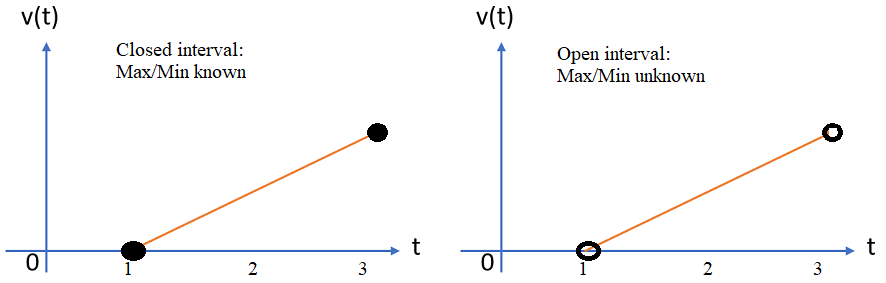# Bounded Interval

A bounded interval (or finite interval) has real numbers for both endpoints. Sequences are bounded if contained within a bounded interval .

• Examples of bounded intervals:
• [0, 1]: Includes both endpoints, 0 and 1,
• [99, 1999]: Includes endpoints 99 and 1999.
• Examples of unbounded intervals:
• (-∞, ∞): does not have a real number for either endpoint,
• The set of all real numbers (ℝ).
• Examples of half-bounded intervals:

Bounded and unbounded intervals can also be closed or open intervals. Open intervals have parentheses and do not include endpoints. Closed intervals, which have brackets, include endpoints. The difference between closed and bounded is that bounded intervals have to have real numbers, while closed intervals can have other numbers, like complex numbers.

## Theorems that Work on a Bounded IntervalA function with a closed interval (left) has a min and max within that interval. But an open interval (right) has problematic endpoint behavior.

Whether or not intervals are bounded rarely crops up as a topic in elementary calculus classes. However, many theorems and procedures only work on these carefully defined intervals. Even if it isn’t explicitly stated in class, working with bounded intervals is implied. For example, all of the following theorems and procedures are defined on bounded intervals:

There are dozens more theorems that only work on a bounded interval.

## References

 Math 401 – Introduction to Real Analysis. Retrieved April 25, 2021 from: http://personal.psu.edu/axb62/PSPDF/lnotes401.pdf
 Additional Topics from 4.2 and 4.3. https://www.math.tamu.edu/~jlewis/131notes/131notes4.2,4.3.pdf
 Part 1: The Real Numbers. Retrieved April 25, 2021 from: https://www.math.uh.edu/~charles/Real-nos.pdf

CITE THIS AS:
Stephanie Glen. "Bounded Interval" From StatisticsHowTo.com: Elementary Statistics for the rest of us! https://www.statisticshowto.com/bounded-interval/